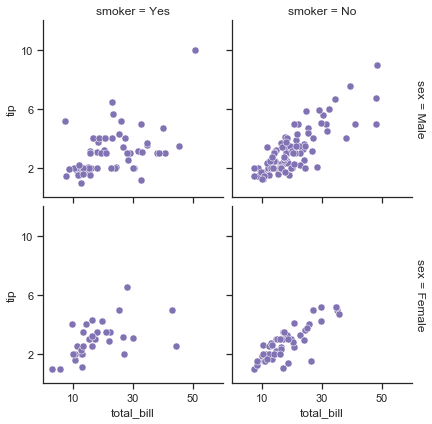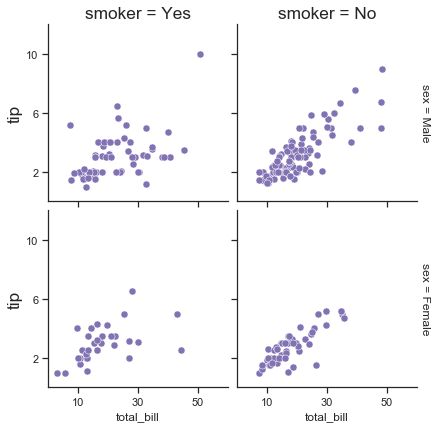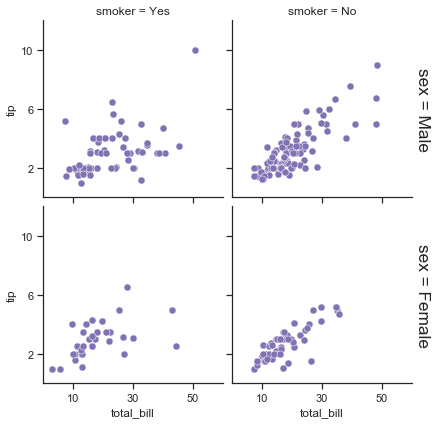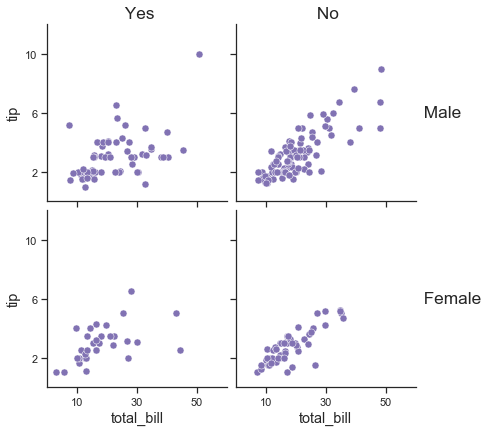# Editing right ylabels in seaborn FacetGrid plots

Published:

Today, I figured out an answer to a question that I didn’t find asked anywhere on the internet. In case someone else (or me) asks this question later, I wanted to write up my solution for reference. This post goes over how to access and manipulate the right y-axis labels on a seaborn FacetGrid plot which was made with `margin_titles = True`.

I’ll just go through one example that the FacetGrid documentation already has, and show how you could go into the axes object to manipulate the right-hand ylabel.

``````import matplotlib.pyplot as plt
import seaborn as sns
sns.set(style="ticks", color_codes=True)
%matplotlib inline

``````

This is the original plot with `margin_titles = True`, using plotting code copied from the doc page and slightly simplified:

``````kws = dict(s=50, linewidth=.5, edgecolor="w")

# Set up the FacetGrid
g = sns.FacetGrid(tips, col="smoker", row="sex",
margin_titles=True)

# Make the plot
g = g.map(plt.scatter, "total_bill", "tip", color="m", **kws)

# Make some changes to the axes, etc (from seaborn documentation)
g.set(xlim=(0, 60), ylim=(0, 12),
xticks=[10, 30, 50], yticks=[2, 6, 10])

``````I figured out a while ago that you can access each individual axes object by calling `g.axes`. This means that you can loop over each axis and use normal calls like `ax.set_title()` and others on each axis. But when I tried this to access the righthand y-axis labels, for example to increase the font size, I wasn’t seeing any difference in the plot:

``````g = sns.FacetGrid(tips, col="smoker", row="sex",
margin_titles=True)
g = g.map(plt.scatter, "total_bill", "tip", color="m", **kws)
g.set(xlim=(0, 60), ylim=(0, 12),
xticks=[10, 30, 50], yticks=[2, 6, 10])

# Iterate thorugh each axis
for ax in g.axes.flat:
ax.set_title(ax.get_title(), fontsize='xx-large')
# This only works for the left ylabels
ax.set_ylabel(ax.get_ylabel(), fontsize='xx-large')
``````I dug around the axes objects quite a bit using various calls to `dir(ax)`, until I found an attribute called `texts` and which seemed to contain the correct text:

``````dir(ax)[390:400]
``````
``````['sticky_edges',
'streamplot',
'table',
'tables',
'text',
'texts',
'tick_params',
'ticklabel_format',
'title',
'titleOffsetTrans']
``````
``````ax.texts
``````
``````[Text(1.02,0.5,u'sex = Female')]
``````

That means that the righthand ylabels are probably just being manually coded in through a call to `ax.text()`, so we can presumably just grab the correct location and text and just change the parameters we want:

``````g = sns.FacetGrid(tips, col="smoker", row="sex",
margin_titles=True)
g = g.map(plt.scatter, "total_bill", "tip", color="m", **kws)
g.set(xlim=(0, 60), ylim=(0, 12),
xticks=[10, 30, 50], yticks=[2, 6, 10])

# Iterate thorugh each axis
for ax in g.axes.flat:

# Only the 2nd and 4th axes have something in ax.texts
if ax.texts:
# This contains the right ylabel text
txt = ax.texts
ax.text(txt.get_unitless_position(), txt.get_unitless_position(),
txt.get_text(),
transform=ax.transAxes,
va='center',
fontsize='xx-large',
rotation=-90)
# Remove the original text
ax.texts.remove()
``````Ta da! Now we know how to access that text and change it to our bidding! We can make it even better (this is how I tend to process my FacetGrid plots for publications):

``````g = sns.FacetGrid(tips, col="smoker", row="sex",
margin_titles=True)
g = g.map(plt.scatter, "total_bill", "tip", color="m", **kws)
g.set(xlim=(0, 60), ylim=(0, 12),
xticks=[10, 30, 50], yticks=[2, 6, 10])

# Iterate thorugh each axis
for ax in g.axes.flat:
# Make x and y-axis labels slightly larger
ax.set_xlabel(ax.get_xlabel(), fontsize='x-large')
ax.set_ylabel(ax.get_ylabel(), fontsize='x-large')

# Make title more human-readable and larger
if ax.get_title():
ax.set_title(ax.get_title().split('='),
fontsize='xx-large')

# Make right ylabel more human-readable and larger
# Only the 2nd and 4th axes have something in ax.texts
if ax.texts:
# This contains the right ylabel text
txt = ax.texts
ax.text(txt.get_unitless_position(), txt.get_unitless_position(),
txt.get_text().split('='),
transform=ax.transAxes,
va='center',
fontsize='xx-large')
# Remove the original text
ax.texts.remove()
``````Tags: